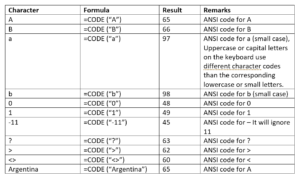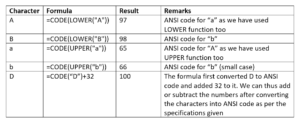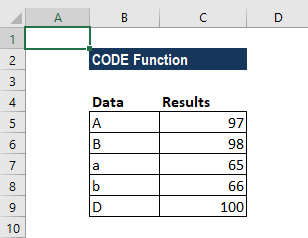# CODE Function

Returns a numeric code for the first character in a given text string

## What is the CODE Function?

The CODE Function is categorized under Excel Text functions. It returns a numeric code for the first character in a given text string. The returned code corresponds to the character set used by the computer. The Windows operating system uses the ANSI character set, whereas Mac OS uses the Macintosh character set.

As a financial analyst, we may not use this function as often as the rest, but we need to understand it if we intend to master Excel functions.

### Syntax

=CODE(text)

The CODE function uses the following argument:

1. Text (required argument) – This is the text for which we want the code of the first character. Excel uses the standard ANSI character set to return the numeric code. If there is more than one character, then it will take the first character and provide the ANSI value for it and ignore the rest of them.

### How to use the CODE Function in Excel?

As a worksheet function, CODE can be entered as part of a formula in a cell of a worksheet.

To understand the uses of the function, let’s consider a few examples:

### Example 1

Let’s see the results we get when we use the CODE function for the data below:The results in Excel are shown below:#### Example 2

We can also use the UPPER and LOWER functions along with the CODE function to get the desired results.

For example, using the data below:The results in Excel are shown below:If we use the function by providing a reference to a cell, it should not be in inverted commas.

### Notes about the CODE Function

1. VALUE# error – Occurs when the text argument is left empty.
2. The result is a numerical value.
3. It was introduced in Excel 2000 and is available in all versions after that.
4. This function is the inverse of the CHAR function.
5. Excel functions CHAR and UNICHAR will return the character for a given code whereas Excel functions CODE and UNICODE will do the opposite – give the code for a given character.

Thanks for reading CFI’s guide to important Excel functions! By taking the time to learn and master these functions, you’ll significantly speed up your financial analysis. To learn more, check out these additional CFI resources:

• Excel Functions for Finance